Home    |    Teacher    |    Parents    |    Glossary    |    About UsDividing is the most challenging of our four basic operations. In fact, you have to use subtraction and multiplication in order to divide, and you also have to be pretty good at rounding and estimating! Many students have trouble with division, perhaps because most problems don't come out nice and even--you really have to use your mental muscle when dividing. Fortunately, we have calculators to make the job easier--but that doesn't mean you shouldn't learn how to do it yourself! It's easy to push a wrong button on the calculator, and you always need to know when the answer it's giving is reasonable.

Dividing decimals is almost the same as dividing whole numbers, except you use the position of the decimal point in the dividend to determine the decimal places in the result.

To divide decimal numbers:

1. If the divisor is not a whole number:
• Move the decimal point in the divisor all the way to the right (to make it a whole number).
• Move the decimal point in the dividend the same number of places.
1. Divide as usual. If the divisor doesn't go into the dividend evenly, add zeroes to the right of the last digit in the dividend and keep dividing until it comes out evenly or a repeating pattern shows up.
2. Position the decimal point in the result directly above the decimal point in the dividend. [Show Me. Show and highlight the decimal point in the quotient, between the 4 and 9]
3. Check your answer: Use the calculator and multiply the quotient by the divisor. Does it equal the dividend?

Let's work through an example.

Find this quotient: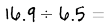First show the division like this: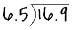Now move the decimal point one place to the right, which makes the divisor a whole number. Also move the decimal point in the dividend one place to the right: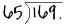Divide as whole numbers. 65 goes into 169 two times with 39 left over: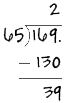To continue dividing, add a zero to the right of the decimal point in the dividend. Then bring down the zero, and add it to the end of 39, making it 390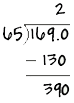65 goes into 390 six times. We write a 6 above the zero in the quotient and put the decimal point just above the decimal point in the dividend: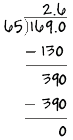To check our answer, we multiply the quotient by the divisor and make sure it equals the dividend: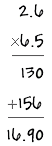Homework Help | Pre-Algebra | NumbersEmail this page to a friendSearch·  Place value·  Decimal numbers·  Estimating and     rounding·  Adding / subtracting     decimals·  Multiplying decimals·  Dividing decimals·  Percent·  Exponents·  Square roots·  Signed integers·  Adding and     subtracting integers·  Multiplying and     dividing integers·  Properties of integersFirst Glance In Depth Examples WorkoutDividing decimals